# An RC circuit as shown in the figure is driven by a AC source generating a square wave.`
Question:

An RC circuit as shown in the figure is driven by a AC source generating a square wave. The output wave pattern monitored by CRO would look close to :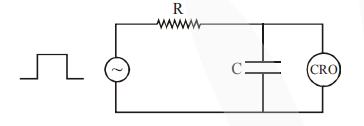1.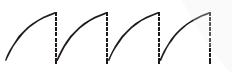2.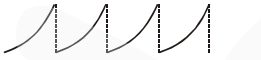3.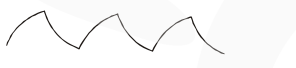4.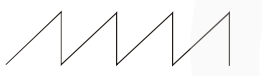Correct Option: , 3

Solution: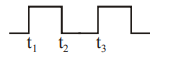For $t_{1}-t_{2}$ Charging graph

$\mathrm{t}_{2}-\mathrm{t}_{3}$ Discharging graph